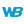Example: Dynamic compilationCreating table columns through programming Compile (Example)See the associated help page Example: Dynamic compilationThe following example is used to dynamically create a procedure according to the choices made by the user. This example is used to enter two digits in two edit controls (EDT_Value1 and EDT_Value2) and to perform a calculation according to the option checked in the radio button. // Example: operation selected in the RADIO_Operation radio buttonRes is stringCodeToCompile is string = "Result1 is int" + CR + ... "Edit2 is int" + CRSWITCH RADIO_Operation CASE 1: // Addition CodeToCompile = CodeToCompile + ... "Result1 = EDT_Value1+EDT_Value2" + CR + ... "Edit2 = EDT_Value1 + EDT_Value2" CASE 2: // Subtraction CodeToCompile = CodeToCompile + ... "Result1 = EDT_Value1-EDT_Value2" + CR + ... "Edit2 = EDT_Value1-EDT_Value2" CASE 3: // Multiplication CodeToCompile = CodeToCompile + ... "Result1 = EDT_Value1*EDT_Value2" + CR + ... "Edit2 = EDT_Value1*EDT_Value2" CASE 4: // Division CodeToCompile = CodeToCompile + ... "Result1 = EDT_Value1/EDT_Value2" + CR + ... "Edit2 = EDT_Value1/EDT_Value2" OTHER CASE: CodeToCompile = ""ENDIF CodeToCompile <> "" THEN CodeToCompile = CodeToCompile + CR + ... "Info(""Result1 = "" + Result1, ""Edit2 = "" + Edit2)" Res = Compile("Calculate", CodeToCompile) IF Res = "" THEN ExecuteProcess("Calculate", trtProcedure) ELSE Error("Error dynamic compilation", Res) ENDELSE Error("No function to compile")END Creating table columns through programmingMyString is string HReadFirst(ZIPCODES, ZipCode)FOR I = 1 TO 5 MyString = MyString + CR +  ... "ControlClone(Column2, """ +  ZIPCODES.City + """)" MyString = MyString + CR +  ... "{""Table1." + ZIPCODES.City + ... """}..Caption = """ + ZIPCODES.City + """" HReadNext(ZIPCODES) END Compile("ColumnCreation", MyString)ExecuteProcess("ColumnCreation", trtProcedure) Minimum version required Version 9 This page is also available for…